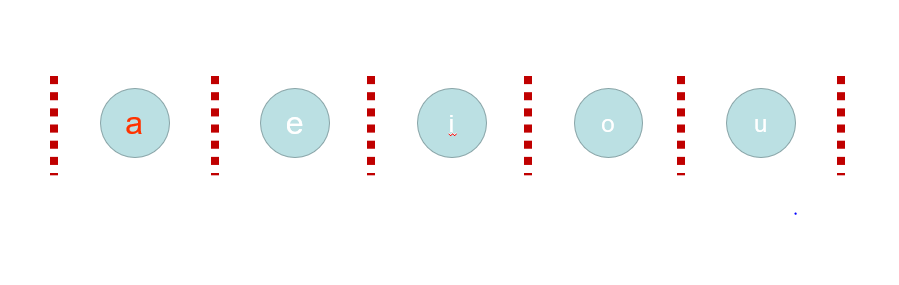By Long Luo

# Math

Every vowel string is start by $a, e, i, o, u$, and there are $N$ strings.

How to distribute $N$ strings into the $5$ boxes, some boxes may be empty?

Imaging that there are $N$ balls, we have to put them in to $5$ boxes represented by the five vowels, as the picture shows.Once the number of characters in each box were fixed, the string is fixed.

Therefore, how many ways are there to put $N$ balls in $5$ boxes, and the boxes can be empty?

The combinatorics explanation is here.

1. $N$ balls in $M$ boxes, box can not empty: $C(n - 1, m - 1)$.

2. $N$ balls in $M$ boxes, box can be empty: $C(n + m - 1, m - 1)$.

## Analysis

• Time Complexity: $O(1)$.
• Space Complexity: $O(1)$.

All suggestions are welcome.
If you have any query or suggestion please comment below.
Please upvote👍 if you like💗 it. Thank you:-)

Explore More Leetcode Solutions. 😉😃💗